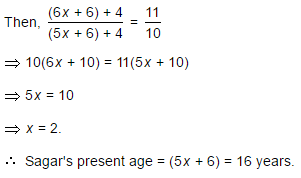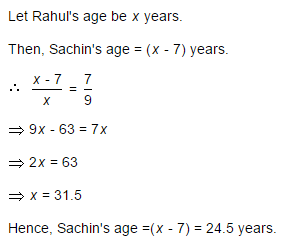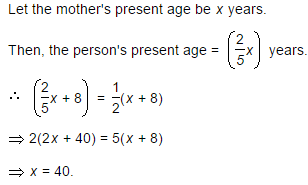Courses

# Test: Problems On Ages - 3

## 10 Questions MCQ Test General Aptitude for GATE 2020 | Test: Problems On Ages - 3

Description
This mock test of Test: Problems On Ages - 3 for Banking Exams helps you for every Banking Exams entrance exam. This contains 10 Multiple Choice Questions for Banking Exams Test: Problems On Ages - 3 (mcq) to study with solutions a complete question bank. The solved questions answers in this Test: Problems On Ages - 3 quiz give you a good mix of easy questions and tough questions. Banking Exams students definitely take this Test: Problems On Ages - 3 exercise for a better result in the exam. You can find other Test: Problems On Ages - 3 extra questions, long questions & short questions for Banking Exams on EduRev as well by searching above.
QUESTION: 1

### A man is 24 years older than his son. In two years, his age will be twice the age of his son. The present age of his son is:

Solution:

Let the son's present age be x years. Then, man's present age = (x + 24) years.(x + 24) + 2 = 2(x + 2)x + 26 = 2x + 4x = 22.

QUESTION: 2

### Six years ago, the ratio of the ages of Kunal and Sagar was 6 : 5. Four years hence, the ratio of their ages will be 11 : 10. What is Sagar's age at present?

Solution:

Let the ages of Kunal and Sagar 6 years ago be 6x and 5x years respectively.QUESTION: 3

### The sum of the present ages of a father and his son is 60 years. Six years ago, father's age was five times the age of the son. After 6 years, son's age will be:

Solution:

Let the present ages of son and father be x and (60 -x) years respectively.

Then, (60 - x) - 6 = 5(x - 6)54 - x = 5x - 306x = 84x = 14.Son's age after 6 years = (x+ 6) = 20 years..

QUESTION: 4

At present, the ratio between the ages of Arun and Deepak is 4 : 3. After 6 years, Arun's age will be 26 years. What is the age of Deepak at present ?

Solution:

Let the present ages of Arun and Deepak be 4x years and 3x years respectively. Then,

4x + 6 = 264x = 20

x = 5.Deepak's age = 3x = 15 years.

QUESTION: 5

Sachin is younger than Rahul by 7 years. If their ages are in the respective ratio of 7 : 9, how old is Sachin?

Solution:QUESTION: 6

The present ages of three persons in proportions 4 : 7 : 9. Eight years ago, the sum of their ages was 56. Find their present ages (in years).

Solution:

Let their present ages be 4x, 7x and 9x years respectively.

Then, (4x - 8) + (7x - 8) + (9x - 8) = 5620x = 80x = 4.Their present ages are 4x = 16 years, 7x = 28 years and 9x = 36 years respectively.

QUESTION: 7

Ayesha's father was 38 years of age when she was born while her mother was 36 years old when her brother four years younger to her was born. What is the difference between the ages of her parents?

Solution:

Mother's age when Ayesha's brother was born = 36 years.

Father's age when Ayesha's brother was born = (38 + 4) years = 42 years.Required difference = (42 - 36) years = 6 years.

QUESTION: 8

A person's present age is two-fifth of the age of his mother. After 8 years, he will be one-half of the age of his mother. How old is the mother at present?

Solution:QUESTION: 9

Q is as much younger than R as he is older than T. If the sum of the ages of R and T is 50 years, what is definitely the difference between R and Q's age?

Solution:

Given that:

1. The difference of age b/w R and Q = The difference of age b/w Q and T.

2. Sum of age of R and T is 50 i.e. (R + T) = 50.

Question: R - Q = ?.

Explanation:

R - Q = Q - T

(R + T) = 2Q

Now given that, (R + T) = 50

So, 50 = 2Q and therefore Q = 25.

Question is (R - Q) = ?

Here we know the value(age) of Q (25), but we don't know the age of R.

Therefore, (R-Q) cannot be determined.

QUESTION: 10

The age of father 10 years ago was thrice the age of his son. Ten years hence, father's age will be twice that of his son. The ratio of their present ages is:

Solution:

Let the ages of father and son 10 years ago be 3x and x years respectively.

Then, (3x + 10) + 10 = 2[(x + 10) + 10]3x + 20 = 2x + 40x = 20.Required ratio = (3x + 10) : (x + 10) = 70 : 30 = 7 : 3.